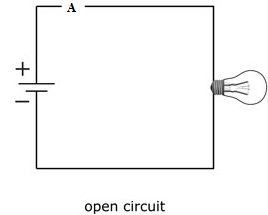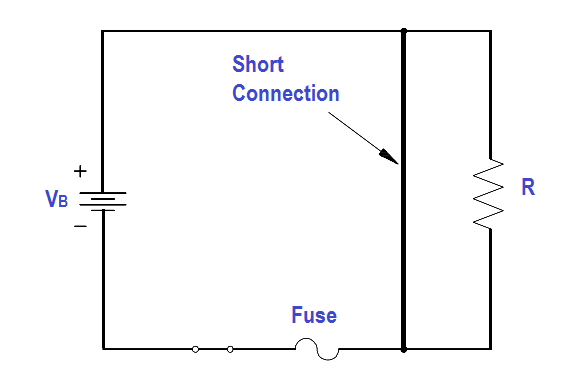### Concept of Open Circuit and Short Circuit

Open Circuit:
The open circuit voltage is nothing but a potential/voltage difference measured between two terminals when no current is flowing through those terminals.• An open circuit is defined as an electric circuit in which current does not flow.

• An open circuit occurs when a circuit is broken from some point.

• An open circuit is where there is no current flow and voltage tends to infinity.

Short Circuit:

• The short circuit current is the tendency of a circuit where current flows when the terminals are forced to have zero voltage/potential difference.• The short circuit occurs when some low resistance path occurs between two points.

• A short circuit is where the voltage tends to zero and current to infinity.